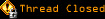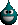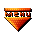# Topic awaiting preservation: Two select boxes and transferring variables (Page 1 of 1)Rane
Bipolar (III) Inmate

From: Denmark
Insane since: Oct 2001posted 11-05-2001 14:40

'afternoon asylum and script-wizardsThings here at work are hot as hell with impossible deadlines etc. - this is why I post this message which I hope you can help me with. Problem:

I have 2 selectboxes - the first one contains predefined values of "1. Intro", "2. Features", "3. Details" etc.etc. The 2nd selectbox should contain other variables depending on what you choose in the first one. Example:

1st box you choose "2. Features" - then the next selectbox should contain "2.1 News", "2.2 Important", "2.3 More info" etc. - which basically means that if you choose a value starting with a "2" in the first box....it should list all the other elements starting with "2." in the 2nd box.

Anyone know how to do that in javascript/dhtml ?

Its to be used in a Lotus Notes web-environment so the values are being extracted from a view in the notes-database - but thats not the main problem here thoWorking code would be greatly appreciated if possible, but snipplets/parts of code may be of use too - as mentioned, we're busy as hell soo...Thanks in advance.lallous
Paranoid (IV) Inmate

From: Lebanon
Insane since: May 2001posted 11-05-2001 15:34

i have made this, hope it helps.

code:
```<html>
<title>New ticket</title>
<meta http-equiv="Content-Type" content="text/html; charset=iso-8859-1">
<script language="JavaScript">
<!--
function init()
{
f = document.myform;
f.f_lbcat.selectedIndex = 0;

f.f_lbcat.options.length = 0;
for (i=0;i<cats.length;i++)
{
temp = String(cats[i]).split('`');
f.f_lbcat.options[i] = new Option(temp, temp, false);
}
f.f_lbcat.onchange();
}

function lbcat_onchange()
{
f = document.myform;
idx = f.f_lbcat.selectedIndex;
if (idx==-1)
idx = 0;
str = cats[idx].split('`');
f.f_lbsubcat.options.length = 0;
len = str.length - 1;
for (i=0;i<len;i++)
{
for (j=1;j<len+1;j++)
{
f.f_lbsubcat.options[j-1] = new Option(str[j], str[j], false);
}
}
}

function checkfields()
{
f = document.myform;
retval = false;
if (f.f_title.value.length==0)
{
}
else if (f.f_body.value.length==0)
{
}
else retval = true;
return retval;
}

//-->
</script>

<script language="JavaScript">
<!--
var cats = new Array(9);
cats = 'Security`SSH`Secure Server`PGP`PGP Mail`Protect Directories';
cats = 'Mail Management`E-mail addresses`Mailing List`News Letter';
cats = 'Development`Script Languages`Java Servlets`Front Page Extensions`Sending Mail';
cats = 'E-commerce`Alacart`Secure Server`Online Payment';
cats = 'Site Management`Site Statistics`FTP`Publishing`Anonymous FTP';
cats = 'Real Audio-Video`Real Server`Video Streaming';

//-->
</script><form name="myform" method="post" action="/hostrix/hib/user/newticket.php" onsubmit="return checkfields();">
<tr>
<td width="159" align="right" height="22">Category:</td>
<td width="489" height="22">
<select name="f_lbcat" onchange="lbcat_onchange();">
</select>
</td>
</tr>
<tr>
<td width="159" align="right">Sub Category:</td>
<td width="489">
<select name="f_lbsubcat">
</select>
</td>
</tr>
<tr>
<td width="159" align="right" height="22">Question Title:</td>
<td width="489" height="22">
<input type="text" name="f_title" size="50">
</td>
</tr>
<tr>
<td width="159" align="right" height="22">Question body:</td>
<td width="489" height="22">
<textarea name="f_body" cols="50" rows="4"></textarea>
</td>
</tr>
<tr>
<td width="159" align="right" height="22"> </td>
<td width="489" height="22">
<input type="submit" name="submit" value="Submit">
<input type="reset" value="Reset">
</td>
</tr>
</table>
</form>
</body>
</html>```

 fixed a small NS! bug[/edit]

[This message has been edited by lallous (edited 11-05-2001).]

lallous
Paranoid (IV) Inmate

From: Lebanon
Insane since: May 2001posted 11-05-2001 16:04

oh yes, here is the PHP snippet that reads the DB and generates the appropriate JS code from the database.

code:
```<script language="JavaScript">
<!--
<?
\$result_c = mysql_query("SELECT * FROM category");
echo "var cats = new Array(" . mysql_num_rows(\$result_c) .");\n";
\$i = 0;
while (\$r_c = mysql_fetch_array(\$result_c))
{
\$result_sc = mysql_query("SELECT * FROM subcategory WHERE catid={\$r_c["id"]}");
\$temp = array();
while (\$r_sc = mysql_fetch_array(\$result_sc))
{
\$temp[] = \$r_sc["name"];
}
\$temp = \$r_c["name"] . "`" . join("`", \$temp);
echo "cats[\$i] = '\$temp';\n";
\$i++;
}
?>```

Rane
Bipolar (III) Inmate

From: Denmark
Insane since: Oct 2001posted 11-06-2001 08:22

Just what I was looking for...thnx lallouslallous
Paranoid (IV) Inmate

From: Lebanon
Insane since: May 2001posted 11-14-2001 11:28

I was reviewing my code again and saw a useless loop, please remove it if you like:

code:
```len = str.length - 1;
[b]for (i=0;i<len;i++)[/b]
{
for (j=1;j<len+1;j++)
{
f.f_lbsubcat.options[j-1] = new Option(str[j], str[j], false);
}
}```

remove the for (i=0;i<len;i++) loop and keep only the for (j=1) loop